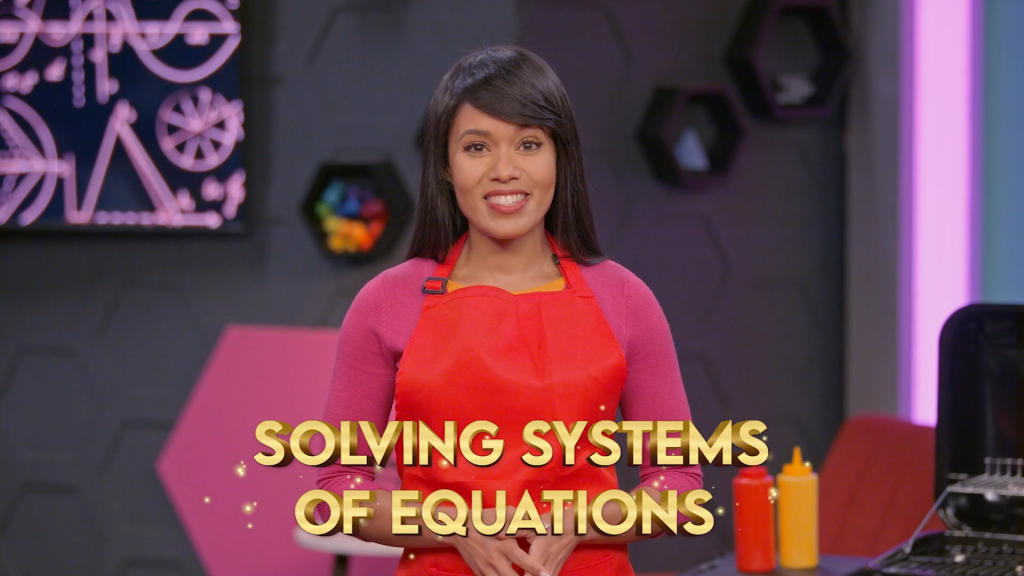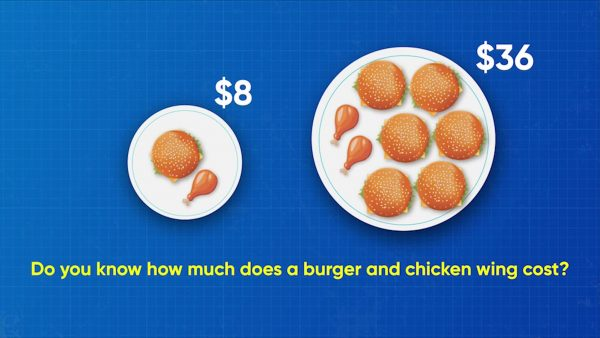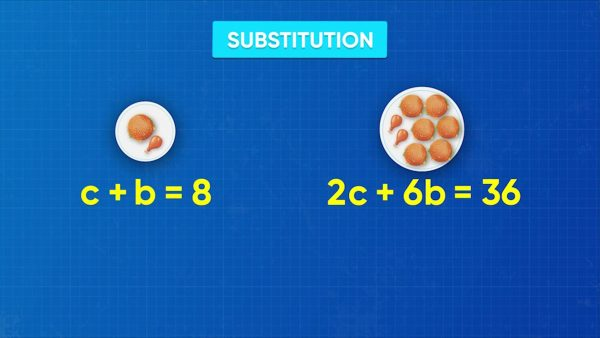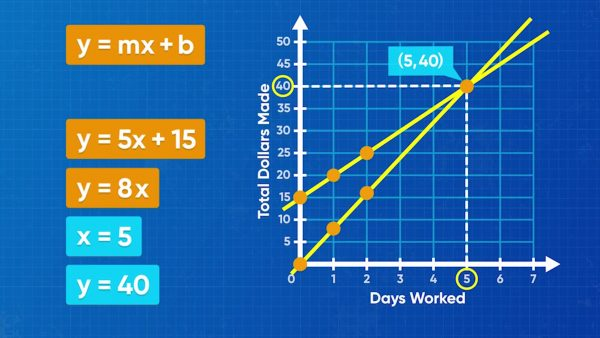Solve Systems of Equations Math Video for Kids - Grades 6-8
1%
It was processed successfully!WHAT IS A SYSTEM OF EQUATIONS?

A system of equations is a set of equations that we need to consider together. A solution to a system of equations is a set of values for the variables that is a solution to every equation in the system.

To better understand systems of equations…

WHAT IS A SYSTEM OF EQUATIONS?. A system of equations is a set of equations that we need to consider together. A solution to a system of equations is a set of values for the variables that is a solution to every equation in the system. To better understand systems of equations…

## LET’S BREAK IT DOWN!

### Make a system of equations to represent BBQ sales.A system of equations is two or more equations that share the same variables. For example: x + y = 5 and 2x + 3y = 8 are two equations that have the same variables, x and y. They are a system of equations. Here is another example. Adesina bought 1 chicken wing and 1 burger for \$8. April bought 6 chicken wings and 2 burgers for \$24. How much do each burger and each chicken wing cost? You can write a system of equations to solve. Let c be the cost of one chicken wing and b be the cost of one burger. Then c + b = 8 and 6c + 2b = 24. These two equations form a system of equations that you can solve to find the answer. Try this yourself: You have some dimes and nickels in a jar. There are 8 coins in all. The total value of the coins is 60 cents. Write a system of linear equations to represent the number of dimes and number of nickels in the jar.

Make a system of equations to represent BBQ sales. A system of equations is two or more equations that share the same variables. For example: x + y = 5 and 2x + 3y = 8 are two equations that have the same variables, x and y. They are a system of equations. Here is another example. Adesina bought 1 chicken wing and 1 burger for \$8. April bought 6 chicken wings and 2 burgers for \$24. How much do each burger and each chicken wing cost? You can write a system of equations to solve. Let c be the cost of one chicken wing and b be the cost of one burger. Then c + b = 8 and 6c + 2b = 24. These two equations form a system of equations that you can solve to find the answer. Try this yourself: You have some dimes and nickels in a jar. There are 8 coins in all. The total value of the coins is 60 cents. Write a system of linear equations to represent the number of dimes and number of nickels in the jar.

### Use substitution to solve for the cost of BBQ goods.You can solve systems of equations using substitution. To do this, rearrange one equation to isolate one variable. Then substitute the value of that variable into the other equation. For the BBQ example, choose either of the two equations to rearrange. Rearranging c+b=8 looks easiest. Pick one variable, and isolate it on one side: b=8-c. Next, replace b with 8-c in the other equation, 6c+2b=24. This gives us 6c+28-c=24. Solve this equation to find the value of c: 6c+16-2c=24, 4c+16=24, 4c=8, and finally c=2. Now we know that a chicken wing costs \$2. We can evaluate one of the original equations at that value to find the other cost. It doesn’t matter which equation we use! Using the first equation, c+b=8, substitute c = 2 and solve for b, which is 6. So, we know that a hamburger costs \$6 and a chicken wing costs \$2. To verify this solution, substitute c = 2 and b = 6 into both equations and check that both sides are equal. Since they are, we know that we have the correct answer. Try this yourself: Solve the system of equations you wrote for the coin problem using substitution.

Use substitution to solve for the cost of BBQ goods. You can solve systems of equations using substitution. To do this, rearrange one equation to isolate one variable. Then substitute the value of that variable into the other equation. For the BBQ example, choose either of the two equations to rearrange. Rearranging c+b=8 looks easiest. Pick one variable, and isolate it on one side: b=8-c. Next, replace b with 8-c in the other equation, 6c+2b=24. This gives us 6c+28-c=24. Solve this equation to find the value of c: 6c+16-2c=24, 4c+16=24, 4c=8, and finally c=2. Now we know that a chicken wing costs \$2. We can evaluate one of the original equations at that value to find the other cost. It doesn’t matter which equation we use! Using the first equation, c+b=8, substitute c = 2 and solve for b, which is 6. So, we know that a hamburger costs \$6 and a chicken wing costs \$2. To verify this solution, substitute c = 2 and b = 6 into both equations and check that both sides are equal. Since they are, we know that we have the correct answer. Try this yourself: Solve the system of equations you wrote for the coin problem using substitution.

### Solve systems of equations by graphing.Emily walks dogs, and she makes \$8 per day. You can represent the money Emily makes using a linear equation with a slope of 8, y=8x, where x represents the number of days worked, and y represents the amount of money she makes. Amari works at the roller-skating rink. He makes \$5 per day, and he already has \$15 saved up. You can represent this with the linear equation y=5x+15, where the 5 shows his daily rate, x represents the number of days worked, and the 15 shows how much he has already saved. You want to find how many days they work before Emily and Amari have the same amount of money. Graph both linear equations on the same coordinate plane. Amari's graph passes through the points (0, 15) and (1, 20). Emily's graph goes through the points (0, 0) and (1, 8). The point where the two lines cross is called the point of intersection, and it is the solution to the system of equations. That is because it is the point that makes both equations true at the same time. This point is (5, 40), so on day 5 both Emily and Amari have \$40. Try this yourself: Graph the lines y=3x+2 and y=4x-2, mark their point of intersection, and estimate its coordinates.

Solve systems of equations by graphing. Emily walks dogs, and she makes \$8 per day. You can represent the money Emily makes using a linear equation with a slope of 8, y=8x, where x represents the number of days worked, and y represents the amount of money she makes. Amari works at the roller-skating rink. He makes \$5 per day, and he already has \$15 saved up. You can represent this with the linear equation y=5x+15, where the 5 shows his daily rate, x represents the number of days worked, and the 15 shows how much he has already saved. You want to find how many days they work before Emily and Amari have the same amount of money. Graph both linear equations on the same coordinate plane. Amari's graph passes through the points (0, 15) and (1, 20). Emily's graph goes through the points (0, 0) and (1, 8). The point where the two lines cross is called the point of intersection, and it is the solution to the system of equations. That is because it is the point that makes both equations true at the same time. This point is (5, 40), so on day 5 both Emily and Amari have \$40. Try this yourself: Graph the lines y=3x+2 and y=4x-2, mark their point of intersection, and estimate its coordinates.

### Many careers require solving systems of equations.Pilots use systems of equations to determine fuel usage and cost for trips. Wildlife rehabilitation centers use systems of equations to determine the cost of caring for different animals. Chemists use systems of equations to calculate the number of ingredients they need for their reactions. Try this yourself: Can you think of how you can use systems of equations in your life or in a career?

Many careers require solving systems of equations. Pilots use systems of equations to determine fuel usage and cost for trips. Wildlife rehabilitation centers use systems of equations to determine the cost of caring for different animals. Chemists use systems of equations to calculate the number of ingredients they need for their reactions. Try this yourself: Can you think of how you can use systems of equations in your life or in a career?

## SOLVE SYSTEMS OF EQUATIONS VOCABULARY

Linear Equation
An equation that, when graphed, is a straight line.
Solution to an equation
A set of values for the variables in the equation that result in a true numeric equation.
System of equations
Two or more equations with the same variables.
Solution to a system of equations
A set of values for the variables in the equations that result in a true numeric equation for all equations in the system.
Substitution method to solve systems of equations
A method for solving systems of equations where one equation is rearranged and substituted into the other equation.
Graphing method to solve systems of equations
A method for solving systems of equations where both equations are graphed on a coordinate plane and the intersection is estimated visually.
The point where two graphs intersect one another, and the solution to a system of equations

## SOLVE SYSTEMS OF EQUATIONS DISCUSSION QUESTIONS

### What is a system of equations?

A system of equations is a set of equations that share all the same variables.

### What is the substitution method?

The substitution method is a method for solving a system of equations. In this method, one equation is set equal to a single variable and then substituted into the other equation.

### What is the graphing method?

The graphing method is a method for estimating the solution to a system of equations. In this method, two linear equations are graphed on the same coordinate plane. The point where the two lines intersect is the solution to the system.

### Does it matter what variable you rearrange for in the substitution method?

No, I get the same answer no matter which variable I rearrange for, or which equation I rearrange first. There may be one variable that is easier to solve for first, in which case I may choose to solve that one first, but I don’t need to.

### When you verify a solution to a system of equations, do you need to check the solution in all the equations in the system?

Yes; it is possible that a point is on one line and not the other. The solution to a system needs to be on both lines, so I need to check both equations.
X

## Success

We’ve sent you an email with instructions how to reset your password.
Ok